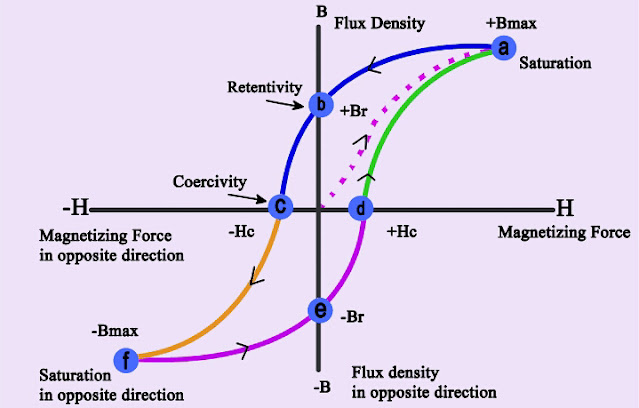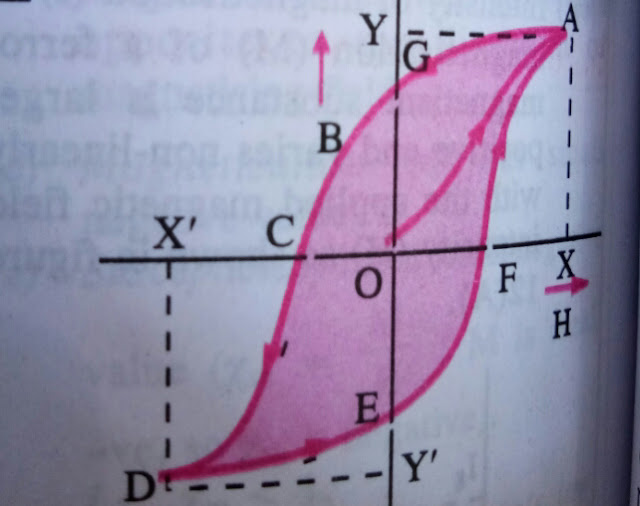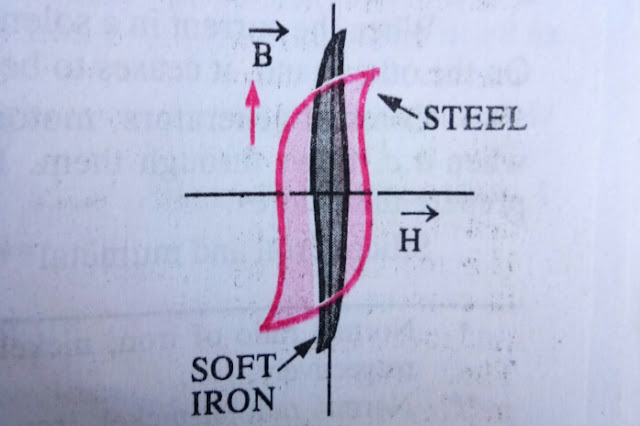# Hysteresis Loop or magnetisation curve - complete information

Views

## Hysteresis Loop or magnetisation curve Introduction:

Hysteresis Loop or magnetisation curve shows the relationship between the induced magnetic flux density (B) and the magnetizing force (H). It is often referred to as the B-H loop. The area of B-H loop is equal to energy per unit volume. Hysteresis is the phenomenon of lagging behind the magnetic induction with respect to the magnetising field.Hysteresis Loop

Magnetisation curve or Hysteresis curve  is the graph between magnetic intensity (H) and magnetic induction (B).

Let's take a solenoid having a Ferromagnetic core inside it, say iron.

### Current in the solenoid is to be increased and decreased In the following steps:Hysteresis Loop

1)   To start with, the iron core is placed in a solenoid having no current. Now, current flowing in the solenoid is increased in steps, so that the magnetic field inside the solenoid increases gradually. This magnetic field is know as magnetising field or magnetic intensity (H) as it magnetises the iron core. As the value of H increases, the magnetic flux density or magnetic induction also increases. The given figure shows the variation between B and H and is represented by curve OA. Further, if we go on increasing the current in the solenoid , it increases the value of H. The value of B which represents magnetic flux density or magnetic field in the iron core does not change.  Thus, point A is known as saturation point corresponding to which B is maximum.

2)  Now, reduce the value of current in the solenoid till the value of H becomes Zero. The iron core begins to demagnetise, placed inside the solenoid that's the value of B decreases along the path AG. when, H=0 , B is not equal to zero but B= OG. It shows that the magnetic field material (say iron core) retains magnetism even if the magnetising field (H)  is reduced to zero. The magnetism retained by the magnetic material even when the magnetising field is reduced to zero is called residual magnetism of the material. The property of the magnetic material to retain magnetism even in the absence of the magnetising field is known as retentivity or remanence.

## Further Steps:

3)   Now,  reverse the direction of flow of the current in the solenoid, so that the magnetising field (H)  acts in the opposite direction (say along negative X-axis). The magnetic field B or the magnetisation of the magnetic material (say iron core) decreases along GC. The magnetic field B becomes zero corresponding to the value of H = OC. This magnetising field (H) needed to completely demagnetise the magnetic material is known as coercivity.

4) Step number four is to increase the value of current in the solenoid in the same direction. This increases the value of H. The value of B also increases in the reverse direction that's along negative Y-axis. In other words, magnetic material  begins to magnetise in the opposite direction till it is completely magnetised. The variation of B with H is represented by the curve CD.

5) The direction of the current is again reversed till the value of H = 0 . Corresponding to H = 0 , the residual magnetism of the material = OE.

6) To completely demagnetise the magnetic material, the current is increased till the magnetic field (B) becomes zero. The material is demagnetised along EF.  On the further increase in the current in the solenoid , value of H increases and hence value of B also increases. The variation of B with H is represented by FA.

The curve AGCDEFA is known as "  Hysteresis Loop " which is the result of a cycle of magnetisation and demagnetisation of the magnetic material.

#### Energy Dissipation due to Hysteresis :

Energy is spent, during the cycle of magnetisation and demagnetisation of a magnetic substance. This energy appears as the heat energy and the substance is heated. Thus, the energy spent is dissipated in the form of heat in the substance. Such energy loss is known as Hysteresis loss. The loss of energy per unit volume of the substance is equal to the area of B-H curve.

#### Importance of the study of Hysteresis loop or curve :

The study of " Hysteresis Loop" of a given substance helps us to know the different properties of the substance. For instance, from the Hysteresis loop of a given material, its retentivity, coercivity, susceptibility, permeability and energy loss per cycle of magnetisation can be calculated.
Therefore, on the basis of these properties, we can select a suitable material or substance for making the cores of generators, electric motors and transformers and also permanent magnets.

#### Illustration :

Hysteresis Loops for soft iron and steel are shown in figure. From the figure, it is clear that ;Hysteresis Loop Illustration

i) The retentivity of soft iron is more than the retentivity of steel.

ii)  Coercivity of soft iron is less than the coercivity of steel. It means, soft iron can be demagnetised easily as compared to the steel

iii) Area of Hysteresis loop of soft iron is much less than the area of the  Hysteresis Loop of steel. Therefore, hysteresis loss in case of steel is greater than that in case of soft iron.

#### Conclusion:

In conclusion  Hysteresis Loop provides the value of  retentivity and coercivity of a material. Thus the way to choose perfect material to make permanent magnet, core of  machines becomes easier.

This article has discussed all the important concepts related to " Hysteresis Loop". Energy dissipation, due to this concept is also discussed. Its importance is also discussed in the article. The article has also illustrated this concept.

1.2.